0
146

Number Series are one of the most important topics in the TISSNET Mathematics section. To ace this topic, one must need to cover all the basics and fundamentals. It is advised to practice a good number of questions from the Number Series. Cracku provides you with some important TISSNET Number Series questions  PDF with detailed solutions. These questions were taken from the previous TISSNET papers, which are very imperative in TISSNET preparation. After solving these questions, one can gain much confidence to solve these types of questions in the actual TISSNET examination. Click on the link below to download the TISSNET Number Series Questions with detailed solutions PDF.

Question 1: Find the missing number in the given series:
8, 13, 10, 15, 12, ____

a) 14

b) 17

c) 18

d) 20

Solution:

we have 8, 13, 10, 15, 12
Here the pattern is 8+5 =13 , 13-3 =10 , 10+5 =15 ;15-3 =12 so next term will be 12+5 =17

Question 2: Select the number that can replace the question mark (?) in the following series.
6, 7, 16, 51, 208, ?

a) 2000

b) 1250

c) 1045

d) 2570

Solution:

The logic here is

$\left(6\times1\right)+1=7$

$\left(7\times2\right)+2=16$

$\left(16\times3\right)+3=51$

$\left(51\times4\right)+4=208$

Similarly, the next number in the series = $\left(208\times5\right)+5=1040+5=1045$

Hence, the correct answer is Option C

Question 3: Select the number that can replace the question mark (?) in the following series.
50, 49, 53, 44, 60, 35, ?

a) 71

b) 117

c) 70

d) 104

Solution:

The given series is 50, 49, 53, 44, 60, 35, ?

49 – 50 = -1

53 – 49 = 4

44 – 53 = -9

60 – 44 = 16

35 – 60 = -25

The difference between the numbers of the series are negative value and positive value of the squares of the consecutive numbers alternatively.

Similarly, the next difference should be 36.

Let the required number be a

$\Rightarrow$  a – 35 = 36

$\Rightarrow$  a = 36 + 35

$\Rightarrow$  a = 71

$\therefore\$The required number is 71.

Hence, the correct answer is Option A

Question 4: Select the number that can replace the question mark (?) in the following series.
6, 10, 18, 34, ?

a) 68

b) 64

c) 66

d) 70

Solution:

The given series is 6, 10, 18, 34, ?

10 – 6 = 4

18 – 10 = 8

34 – 18 = 16

The logic here is the difference between the numbers is twice the previous difference.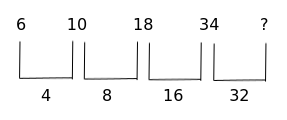The next difference should be 32.

Let the required number be a

$\Rightarrow$  a – 34 = 32

$\Rightarrow$  a = 32 + 34

$\Rightarrow$  a = 66

$\therefore\$The required number is 66.

Hence, the correct answer is Option C

Question 5: Identify the number that does NOT belong to the following series.
1, 4, 9, 15, 25, 36

a) 4

b) 9

c) 15

d) 25

Solution:

The given series is 1, 4, 9, 15, 25, 36

The logic here is

1$^2$ = 1

2$^2$ = 4

3$^2$ = 9

4$^2$ = 16

5$^2$ = 25

6$^2$ = 36

16 should be in the place of 15 in the series

$\therefore\$15 does not belong to the given series

Hence, the correct answer is Option C

Question 6: Select the number that can replace the question mark (?) in the following series.
1, 27, 125, 343, 729, ?

a) 1244

b) 1525

c) 1331

d) 1029

Solution:

The given series is 1, 27, 125, 343, 729, ?

1 = 1$^3$

27 = 3$^3$

125 = 5$^3$

343 = 7$^3$

729 = 9$^3$

Similarly, the next number in the series = 11$^3$ = 1331

$\therefore\$Required number = 1331

Hence, the correct answer is Option C

Question 7: Select the number that can replace the question mark (?) in the following series.
80, 79, 75, 66, ?, 25

a) 46

b) 57

c) 50

d) 54

Solution:

Given series is 80, 79, 75, 66, ?, 25

The logic here is

80 – 79 = 1 = 1$^2$

79 – 75 = 4 = 2$^2$

75 – 66 = 9 = 3$^2$

The difference between the numbers of the series is the square of natural numbers.

The next difference will be 4$^2$ = 16.

Let the required number = a

$\Rightarrow$  66 – a = 16

$\Rightarrow$  a = 50

$\therefore\$Required number = 50

Hence, the correct answer is Option C

Question 8: Select the number that can replace the question mark (?) in the following series.
19, 20, 24, 51, 67, ?

a) 190

b) 192

c) 191

d) 195

Solution:

In the given series,

20 – 19 = 1 = 1$^3$

24 – 20 = 4 = 2$^2$

51 – 24 = 27 = 3$^3$

67 – 51 = 16 = 4$^2$

The difference between numbers of the series are square and cubes of natural numbers alternatively.

The next difference should be 5$^3$

Let the required number be ‘a’

$\Rightarrow$  a – 67 = 5$^3$

$\Rightarrow$  a = 125 + 67

$\Rightarrow$  a = 192

$\therefore\$Required number = 192

Hence, the correct answer is Option B

Question 9: Identify the number that does NOT belong to the following series.
81, 54, 36, 27, 22

a) 27

b) 36

c) 22

d) 54

Solution:

81 – 54 = 27

54 – 36 = 18

36 – 27 = 9

The difference of consecutive numbers is the decreasing multiple of 9. The next difference should be 0.

Similarly, the next number in the series = 27 + 0 = 27

The required series should be 81, 54, 36, 27, 27

$\therefore\$22 do not belong to the series.

Hence, the correct answer is Option C

Question 10: Select the number that can replace the question mark (?) in the following series.
2, 3, 6, 15, ?, 157.5, 630

a) 54

b) 49

c) 48

d) 45

Solution:

Given series is 2, 3, 6, 15, ?, 157.5, 630

The logic here is

2 x 1.5 = 3

3 x 2 = 6

6 x 2.5 = 15

Similarly, the next number = 15 x 3 = 45

45 x 3.5 = 157.5

157.5 x 4 = 630

$\therefore\$The required number = 45

Hence, the correct answer is Option D

Question 11: Select the number that can replace the question mark (?) in the following series.
8, 13, 23, 43, ?, 163, 323

a) 79

b) 63

c) 83

d) 93

Solution:

The given series is 8, 13, 23, 43, ?, 163, 323

The difference of the numbers is becoming twice as shown below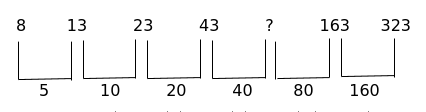The next difference should be 40.

Let the required number = a

$\Rightarrow$ a – 43 = 40

$\Rightarrow$ a = 83

$\therefore\ \$Required number = 83

Hence, the correct answer is Option C

Question 12: Select the number that can replace the question mark (?) in the following series.
2, 8, 15, 24, 36, 52, ?

a) 83

b) 73

c) 78

d) 63

Solution:

Given series is 2, 8, 15, 24, 36, 52, ?

The logic here is difference of differences of the numbers are consecutive natural numbers as shown below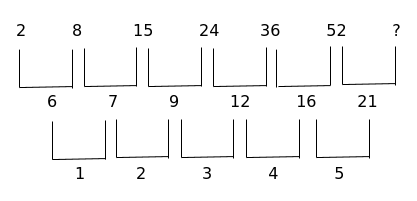The next natural number will be 5 and the difference between required number and 52 should be 21.

$\therefore\$Required number = 52 + 21 = 73

Hence, the correct answer is Option B

Question 13: Select the number that can replace the question mark (?) in the following series.
25, 5, 49, 10, 81, 20, 121, ?

a) 30

b) 40

c) 35

d) 25

Solution:

The given series is a alternate series with the two series are 25, 49, 81, 121, …  and  5, 10, 20, …

The next term of the given series is the next term of 5, 10, 20, …

In the series 5, 10, 20, ….

5 x 2 = 10

10 x 2 = 20

Number in the series is twice the previous number.

Similarly, the next number = 20 x 2 = 40

$\therefore\$Next number of the given series is 40

Hence, the correct answer is Option B

Question 14: Select the number that can replace the question mark (?) in the following series.
10, 26, 50, 82, ?

a) 121

b) 101

c) 169

d) 122

Solution:

The logic here is

26 – 10 = 16

50 – 26 = 24

82 – 50 = 32

The difference between the numbers of the series is multiple of 8

The next difference should be 40

$\therefore\$Required number = 82 + 40 = 122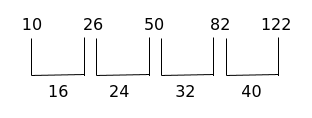Hence, the correct answer is Option D

Question 15: Select the number that can replace the question mark (?) in the following series.
2, 10, 26, ?, 82

a) 65

b) 58

c) 50

d) 46

Solution:

The logic here is

10 – 2 = 8

26 – 10 = 16

The difference between the numbers of the series is multiple of 8

The next difference should be 24

$\therefore\$Required number = 26 + 24 = 50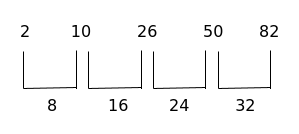Hence, the correct answer is Option C

Question 16: Select the number that can replace the question mark (?) in the following series.
126, 217, 344, ?

a) 513

b) 521

c) 729

d) 512

Solution:

126 = 125 + 1 = 5$^3$ + 1

217 = 216 + 1 = 6$^3$ + 1

344 = 343 + 1 = 7$^3$ + 1

The number of the series are in the form of n$^3$ + 1

$\therefore\$Next number in the series = 8$^3$ + 1 = 513

Hence, the correct answer is Option A

Question 17: Select the number that can replace the question mark (?) in the following series.
213, 217, 233, 269, ?

a) 296

b) 333

c) 428

d) 312

Solution:

217 – 213 = 4 = 2$^2$

233 – 217 = 16 = 4$^2$

269 – 233 = 36 = 6$^2$

The difference between the consecutive numbers of the series are squares of even numbers.

The next difference is 8$^2$ = 64

Let the required number = a

$\Rightarrow$ a – 269 = 64

$\Rightarrow$ a = 333

$\therefore\$The required number = 333

Hence, the correct answer is Option B

Question 18: Select the number that can replace the question mark (?) in the following series.
5, 3, 3, 5, 15, ?

a) 59

b) 45

c) 69

d) 39

Solution:

The logic here is

5 x 1 – 2 = 3

3 x 2 – 3 = 3

3 x 3 – 4 = 5

5 x 4 – 5 = 15

Similarly, the next number in the series = 15 x 5 – 6 = 69

Hence, the correct answer is Option C

Question 19: Select the number that can replace the question mark (?) in the following series.
5, 5, 15, 75, 525, ?

a) 1575

b) 2625

c) 4725

d) 1050

Solution:

The logic here is

5 x 1 = 5

5 x 3 = 15

15 x 5 = 75

75 x 7 = 525

Numbers of the series are multiplied by consecutive odd number.

Similarly, the next number in the series = 525 x 9 = 4725

Hence, the correct answer is Option C

Question 20: Select the number that can replace the question mark (?) in the following series.
7, 16, 24, 31, 37, ?

a) 45

b) 39

c) 42

d) 58

Solution:

The logic here is

7 + 9 = 16

16 + 8 = 24

24 + 7 = 31

31 + 6 = 37

Similarly, the next number in the series = 37 + 5 = 42

Hence, the correct answer is Option C